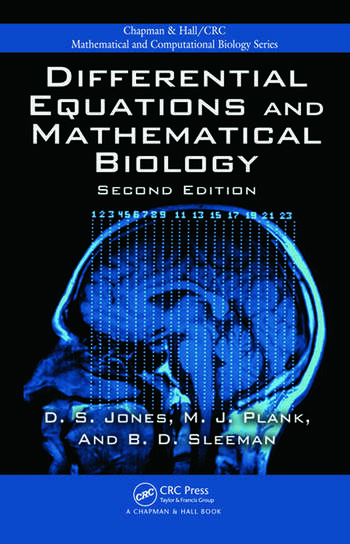Differential Equations and Mathematical Biology

2nd Edition

D.S. Jones, Michael Plank, B.D. Sleeman

Chapman and Hall/CRC
Published November 9, 2009
Textbook - 462 Pages - 100 B/W Illustrations
ISBN 9781420083576 - CAT# C8357
Series: Chapman & Hall/CRC Mathematical and Computational Biology

For Instructors Request Inspection Copy

was \$98.95

USD\$79.16

SAVE ~\$19.79

FREE Standard Shipping!

Preview

Summary

Deepen students’ understanding of biological phenomena

Suitable for courses on differential equations with applications to mathematical biology or as an introduction to mathematical biology, Differential Equations and Mathematical Biology, Second Edition introduces students in the physical, mathematical, and biological sciences to fundamental modeling and analytical techniques used to understand biological phenomena. In this edition, many of the chapters have been expanded to include new and topical material.

New to the Second Edition

• A section on spiral waves
• Recent developments in tumor biology
• More on the numerical solution of differential equations and numerical bifurcation analysis
• MATLAB® files available for download online
• Many additional examples and exercises

This textbook shows how first-order ordinary differential equations (ODEs) are used to model the growth of a population, the administration of drugs, and the mechanism by which living cells divide. The authors present linear ODEs with constant coefficients, extend the theory to systems of equations, model biological phenomena, and offer solutions to first-order autonomous systems of nonlinear differential equations using the Poincaré phase plane. They also analyze the heartbeat, nerve impulse transmission, chemical reactions, and predator–prey problems. After covering partial differential equations and evolutionary equations, the book discusses diffusion processes, the theory of bifurcation, and chaotic behavior. It concludes with problems of tumor growth and the spread of infectious diseases.

Instructors

We provide complimentary e-inspection copies of primary textbooks to instructors considering our books for course adoption.